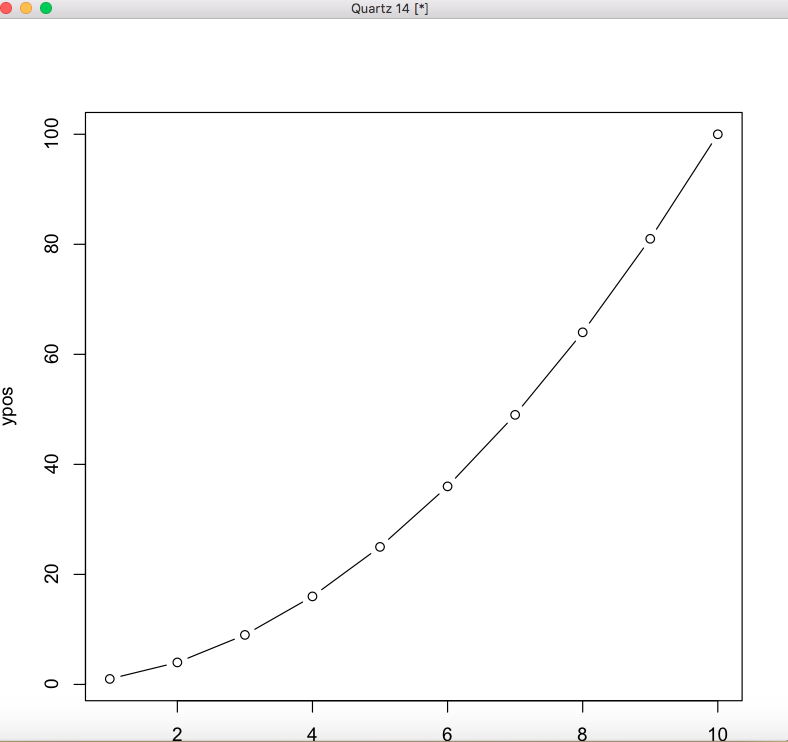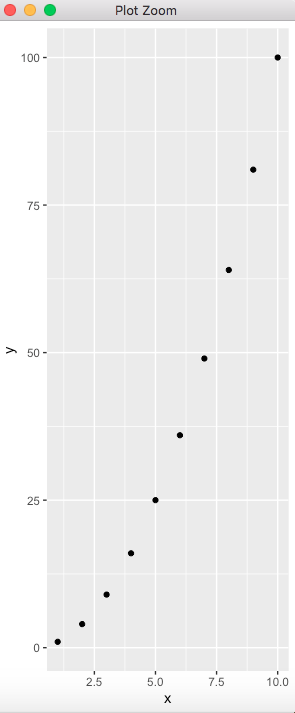# Export Plot to EPS File in R

An EPS file is a graphics file saved in the Encapsulated PostScript (EPS) file format. The EPS files may contain images, graphics, or even text. In this article, we will discuss how we export a plot to an EPS file using R programming language.

### Method 1 : Using setEPS() method

The postscripts can be defined using the setEPS() method in R. postscript initiates the graphics device driver for producing PostScript graphics. The eps file is stored in the current working directory by default. To save it to a specific location specify the full destination path along with the name of the file in postscript() function.

Example:

## R

 `# declaring the xpos vector  ` `xpos <- ``c``(1:10) ` ` `  `# declaring the ypos vector  ` `# equivalent to x^2 ` `ypos <- xpos^2 ` ` `  `setEPS``() ` ` `  `# naming the eps file ` `postscript``(``"gfg.eps"``) ` ` `  `# plotting the x and y position ` `# vectors ` `plot``(xpos,ypos) ` `dev.off``() `

Output### Method 2: Using ggplot2

The ggplot library can be installed and loaded into the working space to work with graphics.The ggplot() method in this package is used to visualize data in the form of scatter plots, boxplots, and time series plots. This method is fed the data in the form of data points, and then a scatter plot is displayed. In this function, an aesthetic mapping(aes) is deployed by selecting the variables x and y and further customized by the addition of a graphical representation of data in the form of points using the geom_point() method in the graph. The plot can be saved in the eps format using the ggplot.save() method, which takes as argument the string name of the plot.

Example:

## R

 `# loading the required libraries ` `library``(``"ggplot2"``) ` ` `  `# declaring the xpos vector  ` `xpos <- 1:10 ` ` `  `# declaring the ypos vector equivalent  ` `# to x^2 ` `ypos <- xpos^2 ` ` `  `# declaring the data using x and y  ` `# position vectors ` `data_frame <- ``data.frame``(x = xpos,        ` `                   ``y = ypos) ` `# plotting the data ` `ggplot``(data_frame, ``aes``(x, y)) + ``geom_point``() ` ` `  `# save the eps plot ` `ggplot.save``(``"gfg.eps"``)`

OutputWhether you're preparing for your first job interview or aiming to upskill in this ever-evolving tech landscape, GeeksforGeeks Courses are your key to success. We provide top-quality content at affordable prices, all geared towards accelerating your growth in a time-bound manner. Join the millions we've already empowered, and we're here to do the same for you. Don't miss out - check it out now!